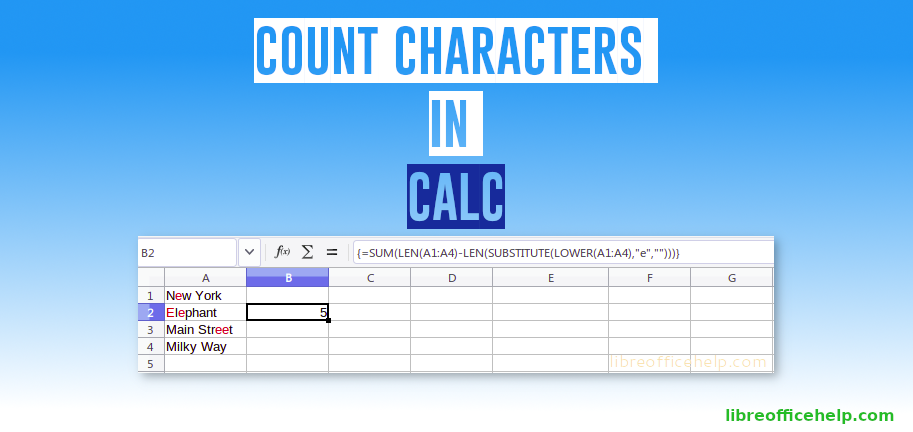## Count Characters in LibreOffice Calc Cell

This beginner’s tutorial explains different ways of counting characters in LibreOffice Calc.

Counting characters in a cell, sheet, range of cells is often useful when doing certain activities. Counting characters very simple in Calc using various functions. Here’s how.

## Count Number of Characters in a Cell

To count number of characters in a Cell, use LEN() function. This function returns the length of a string inside a cell including spaces.

## Count Number of Characters in a Range of Cells

You can count the characters in a range of cells in total using SUM() and LEN() function together.

However, the problem with above formula is it can become very very long as you try to count more cells. In those cases you can use Array formula. Array formula are a way of defining cells for individual calculations. Array formula is applied in any cell in Calc using CTRL+SHIFT+ENTER key combination (see below). Once you apply said combination, Calc adds curly braces {} to the array formula.

## Count Occurrences of a specific character in a Cell

You can combine LEN() function and SUBSTITUTE() function in Calc to count the occurrence of a specific character in a cell. SUBSTITUTE() function takes three arguments –

SUBSTITUTE(target string, search char, replaced by char)

You can replace any character with empty character and subtract length of cell after replacement from total length to find out the occurrence of a character. For example, below formula returns 2 which counts “e” in cell A3 which contains “Main Street”.

## Count Occurrences of a specific character in a range of cells

Using the previous method, you can count the occurrence of a single character in a range of cells using array function. Below example counts number of “e” in A1:A4 range. Note that it only counted small letter “e” and skipped the capital letter “E”.